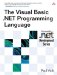# Integer Data Types

 <  Day Day Up  >

The integer data types store numbers with no fractional part. Because processing integers is simpler than processing numbers that have fractional parts , in most cases working with integer types will be faster than working with decimal numbers . The four integer types have different sizes and ranges.

• The Byte data type is one byte long and is the smallest of the integer data types. Its range is from 0 to 255, and it cannot represent negative numbers.

• The Short data type is two bytes long. Its range is from “32,768 to 32,767.

• The Integer data type is four bytes long. Its range is from “2,147,483,648 through 2,147,483,647.

• The Long data type is eight bytes long. Its range is from “9,223,372,036,854,775,808 through 9,223,372,036,854,775,807.

Because of the way that signed integer types are represented in the .NET Framework, Short , Integer , and Long have a range that is one smaller on the positive side than it is on the negative side (i.e., the minimum value of Short is “32,768, but the maximum value is only 32,767)

## Compatibility

The ranges of the Integer and Long types have been widened from previous versions, and a new type, Short , has been added. The range of Short is the same as the range of Integer in previous versions, and the range of Integer is equivalent to the range of Long in previous versions. When you are converting code from previous versions of Visual Basic, it is important to keep in mind this difference.

By default, most programs should use the Integer type to store integer numbers because it is the most natural integer size on the .NET Framework. For numbers that have a larger range than Integer , Long is an acceptable choice. The Byte and Short data types are less efficient on the Framework than Integer and Long , and so should not be used unless there are space concerns (such as may be the case with large arrays of integer values). The default value of the integer types is the literal .

Byte and Short tend to be less efficient at runtime because the Framework only represents numbers as 32-bit or 64-bit values. Thus, when you are performing operations on Byte and Short values, additional overflow checks must be explicitly performed to ensure that the resulting value stays within the range of the declared type. Nevertheless, their storage space savings may justify their use, especially in large arrays.

#### Integer Literals

Integer literals can be specified using one of three bases: decimal, octal, and hexadecimal. Decimal integer literals are decimal (base 10) numbers such as 10 , 43274 , and 34920492 . Octal integer literals are preceded by an ampersand ( & ) and the letter O, and are made up of a string of octal (base 8) digits, such as &O30 , &O4123 , and &O7372 . Hexadecimal integer literals are preceded by an ampersand and the letter H, and are made up of a string of hexadecimal (base 16) digits ”the numbers 0 “9 and the letters A “F ”such as &H3A , &H49D , and &H4932 . The following shows some examples of integer literals.

` Dim a As Byte Dim b As Short Dim c As Integer Dim d As Long a = 10 b = &O43S c = 32767 d = &HFFFFFFFFFL `

Decimal literals represent the decimal value of the literal, while octal and hexadecimal literals represent the binary value of the literal. In the preceding example, the hexadecimal literal &HFFFFFFFFL is equal to the decimal literal -1L .

The type of an integer literal is always Integer unless it does not fit into the range of Integer . In that case, the type of the integer literal is Long .

Integer literals are not signed. As a result, the expression -10 is interpreted as the negation operator applied to the integer literal 10 . This has no consequence in most situations except when two particular values are being interpreted. The expression “ 2147483648 is typed as Long instead of Integer , because the literal 2147483648 will not fit inside an Integer type (even though its negative will). Similarly, the expression “ 9223372036854775808 will overflow because the literal 9223372036854775808 will not fit inside a Long type (even though its negative will).

An integer literal can also be explicitly typed by following the literal with a type character: S for Short , I for Integer , and L for Long . There is no type character for Byte , because it would be ambiguous when used with a hexadecimal literal (i.e., &H1B ).

## Style

The characters % and & can be used as type characters for Integer and Long , respectively, but their inclusion is for historical reasons, and their use is discouraged.

 <  Day Day Up  >The Visual Basic .NET Programming Language
ISBN: 0321169514
EAN: 2147483647
Year: 2004
Pages: 173
Authors: Paul Vick

Similar book on Amazon